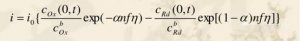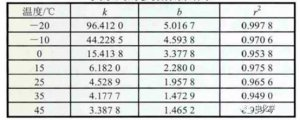# How current affects battery internal resistance?

The internal resistance of the cell includes ohmic internal resistance and polarization of internal resistance. Generally，the ohmic internal resistance of a battery is caused by processes such as electrolyte ion diffusion and ions passing through the diaphragm and has little to do with the current rate, while the polarization internal resistance is caused by the charge transfer process of the electric double layer, which is very much related to the current rate.  Many existing documents generally do not consider the relationship between the battery internal resistance and the current rate when modeling the battery. This is because the charge and discharge current rate of the battery is not large, and the battery internal resistance can simply be considered as the basic change with the rate. constant. However, when the battery outputs the maximum power, the discharge rate of the battery may be as high as 3 C. At this time, the influence of the current rate on the internal resistance of the battery cannot be ignored. Therefore, when using the battery model to calculate the battery’s limit discharge power, the change rule of the battery’s internal resistance with the current rate must be considered, otherwise, the battery’s limit discharge power cannot be accurately estimated.

According to the electrochemical theory, the internal polarization resistance of the battery is caused by the charge transfer process of the electric double layer composed of SEI film, etc.In the formula: i is the current density, η is the overpotential, α=0.5, cOx is the oxide concentration, and cRd is the reductant concentration. When the overpotential η is very large, the above equation can be simplified to the following equation.The formula for calculating the internal polarization resistance of the battery is shown in formula (7). Among them, b(i) is related to the solid phase liquid phase lithium ion concentration distribution inside the lithium ion, and these concentration distributions are related to the current density i. When the current density i is large, for the sake of simplicity, consider that b(i) is proportional to i, and then the polarization internal resistance of the battery can be considered to have a linear relationship with ln(i)/i, as shown in the following equation. Furthermore, the battery internal resistance includes ohmic internal resistance and polarization of internal resistance. The ohmic internal resistance can be considered to be independent of the rate, and the battery internal resistance also has a linear relationship with ln(i)/i.The figure below shows the fitting curve of battery internal resistance and current ln (I )/I when SOC = 0.6 at different temperatures. It can be seen that as the current I increases, ln(I )/I decreases, The internal resistance of the battery also decreases, showing a good linear relationship with ln(I)/I. The table lists the fitting results of the relationship curves at different temperatures in the figure. It can be seen that the fitting degree r2 is higher than 0.94, and the fitting degree is very good, which verifies the results derived from the formula in the article.When SOC=0.6, the fitting results of battery internal resistance and discharge rate at different temperaturesAbove, based on electrochemical principles, it proposes a new relationship between battery internal resistance and current rate, that is, battery internal resistance R and ln(I)/I have a linear relationship, which is verified by experimental results.